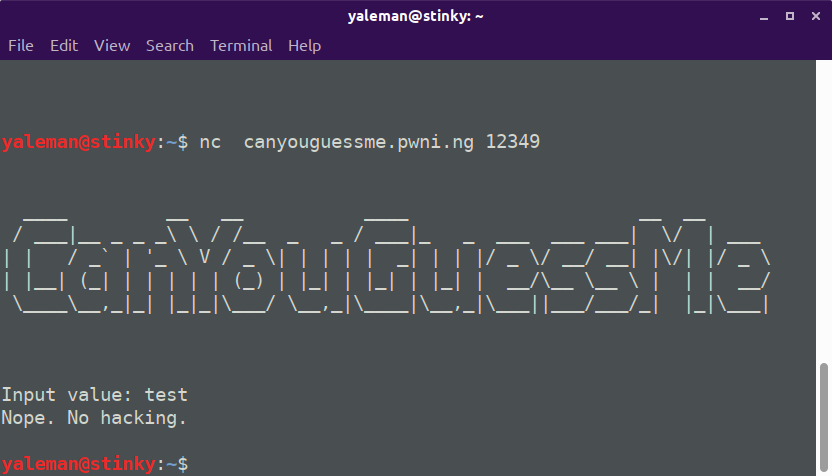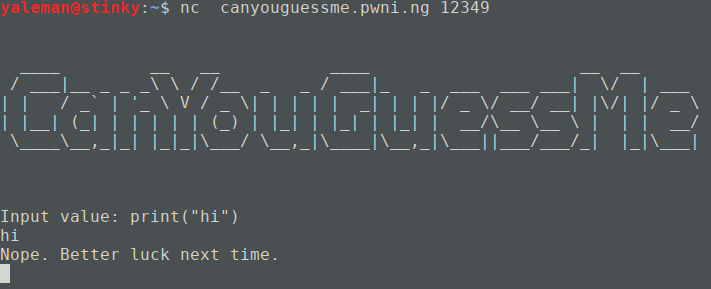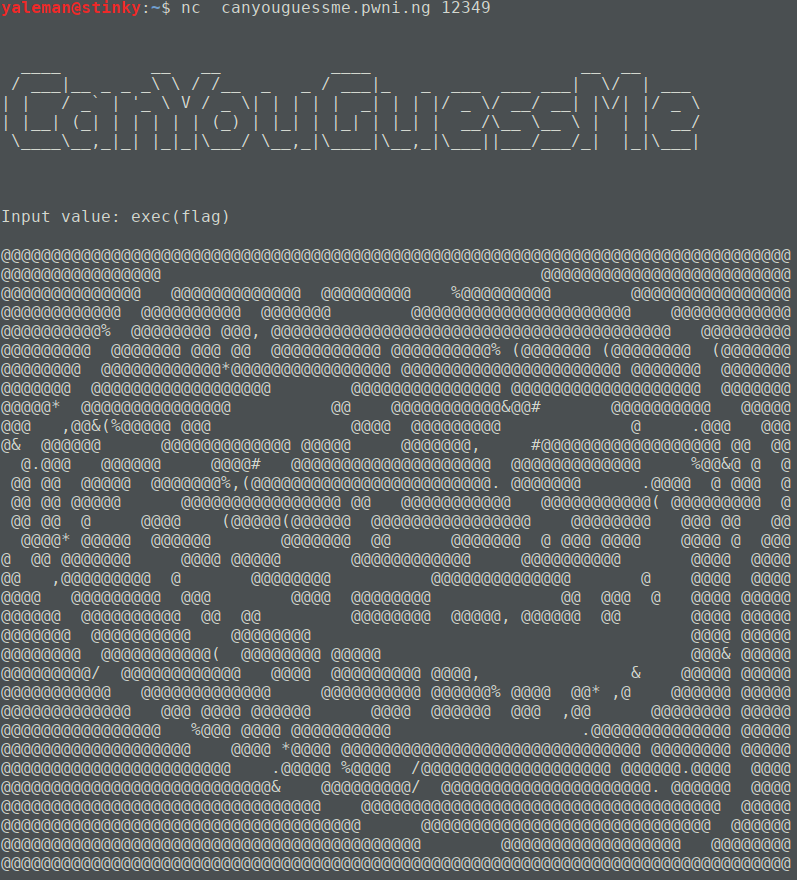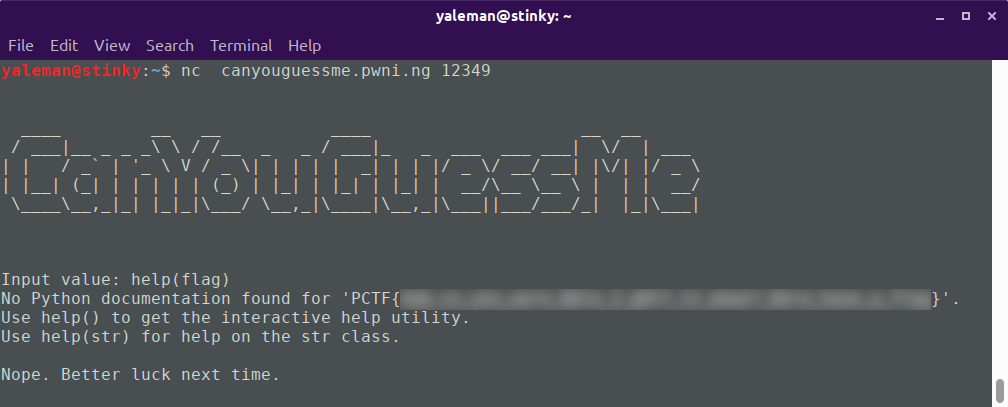PlaidCTF - Can You Guess Me

A friend asked me for help with this one. I hadn’t planned on doing the Plaid CTF but I’m easily dragged into a neat programming challenge.

can you guess me

Misc (100 pts)

Here’s the source to a guessing game: here

You can access the server at `nc canyouguessme.pwni.ng 12349`Nothing ridiculously simple here, the solution’s obviously in the code… here’s the code that was provided:

``````#! /usr/bin/env python3

from sys import exit
from secret import secret_value_for_password, flag, exec

print(r"")
print(r"")
print(r"  ____         __   __           ____                     __  __       ")
print(r" / ___|__ _ _ _\ \ / /__  _   _ / ___|_   _  ___  ___ ___|  \/  | ___  ")
print(r"| |   / _` | '_ \ V / _ \| | | | |  _| | | |/ _ \/ __/ __| |\/| |/ _ \ ")
print(r"| |__| (_| | | | | | (_) | |_| | |_| | |_| |  __/\__ \__ \ |  | |  __/ ")
print(r" \____\__,_|_| |_|_|\___/ \__,_|\____|\__,_|\___||___/___/_|  |_|\___| ")
print(r"                                                                       ")
print(r"")
print(r"")

try:
val = 0
inp = input("Input value: ")
count_digits = len(set(inp))
if count_digits <= 10:          # Make sure it is a number
val = eval(inp)
else:
raise

print(flag)
else:
print("Nope. Better luck next time.")
except:
print("Nope. No hacking.")
exit(1)
``````

Pretty simple so far, it’s got a few imports, allows you to type something in, does a bit of a check and if you do things right, it’ll show you the flag.

Initial observations:

• Imports three things from a `secret` package; `secret_value_for_password, flag, exec`.
• It’s using exception handling for when we’re sneaky.
• The user can enter arbitrary text.
• It’ll “make sure it’s a number”.
• If not, then throw an exception which byasses the rest of the code.
• If it is, then eval() it - so we can run arbitrary python code!
• If the return value of `eval(inp)` is the “secret password”, print the flag.

Now this is where we went wrong initially, focusing on the “number” part - it’s not numbers at all - it’s just a furphy. The value of `len(set(inp))` is the count (len) of unique characters (set) in the input - our main limitation.

For example:

• `set('test') = ['t', 'e', 's' ]` - length 3
• `set('aaa') = ['a']` - length 1

So what we can enter is any combination of code as long as we don’t use more than ten unique characters. I’m a lazy programmer, so I played around a little in the REPL:

``````>>> def lenset(text):
...   return len(set(text))
...
>>> lenset('test')
3
>>> lenset('aaa')
1
>>> lenset('print("hi")')
9
``````hrm.

``````>>> lenset('print(secret_value_for_password)')
19
>>> lenset('print(flag)')
11
>>> lenset('exec(flag)')
9
``````

Oooooh.Bleh.

Suffice it to say, a bunch of variations on this didn’t work. Neither did printing anything, exec’ing random things - remembering that any exception bins the connection, and anything to do with the secret value was clearly way too long.

I was trying to remember every built-in python function that had a short name…

``````>>> [ b for b in dir(__builtins__) if len(set(b)) <= 8 ]
['BufferError', 'EOFError', 'Ellipsis', 'False', 'IOError', 'ImportError', 'IndexError', 'KeyError', 'LookupError', 'MemoryError', 'NameError', 'None', 'OSError', 'ReferenceError', 'TabError', 'True', 'TypeError', 'ValueError', 'Warning', '_', '__debug__', '__doc__', '__import__', '__loader__', '__name__', '__package__', '__spec__', 'abs', 'all', 'any', 'ascii', 'bin', 'bool', 'bytearray', 'bytes', 'callable', 'chr', 'compile', 'complex', 'credits', 'delattr', 'dict', 'dir', 'divmod', 'enumerate', 'eval', 'exec', 'exit', 'filter', 'float', 'format', 'frozenset', 'getattr', 'globals', 'hasattr', 'hash', 'help', 'hex', 'id', 'input', 'int', 'isinstance', 'issubclass', 'iter', 'len', 'license', 'list', 'locals', 'map', 'max', 'memoryview', 'min', 'next', 'object', 'oct', 'open', 'ord', 'pow', 'print', 'property', 'quit', 'range', 'repr', 'reversed', 'round', 'set', 'setattr', 'slice', 'sorted', 'str', 'sum', 'super', 'tuple', 'type', 'vars', 'zip']
``````

So many to choose from… I can skip all the errors, variable manipulation and so forth… and that basically leaves help.Blerp!